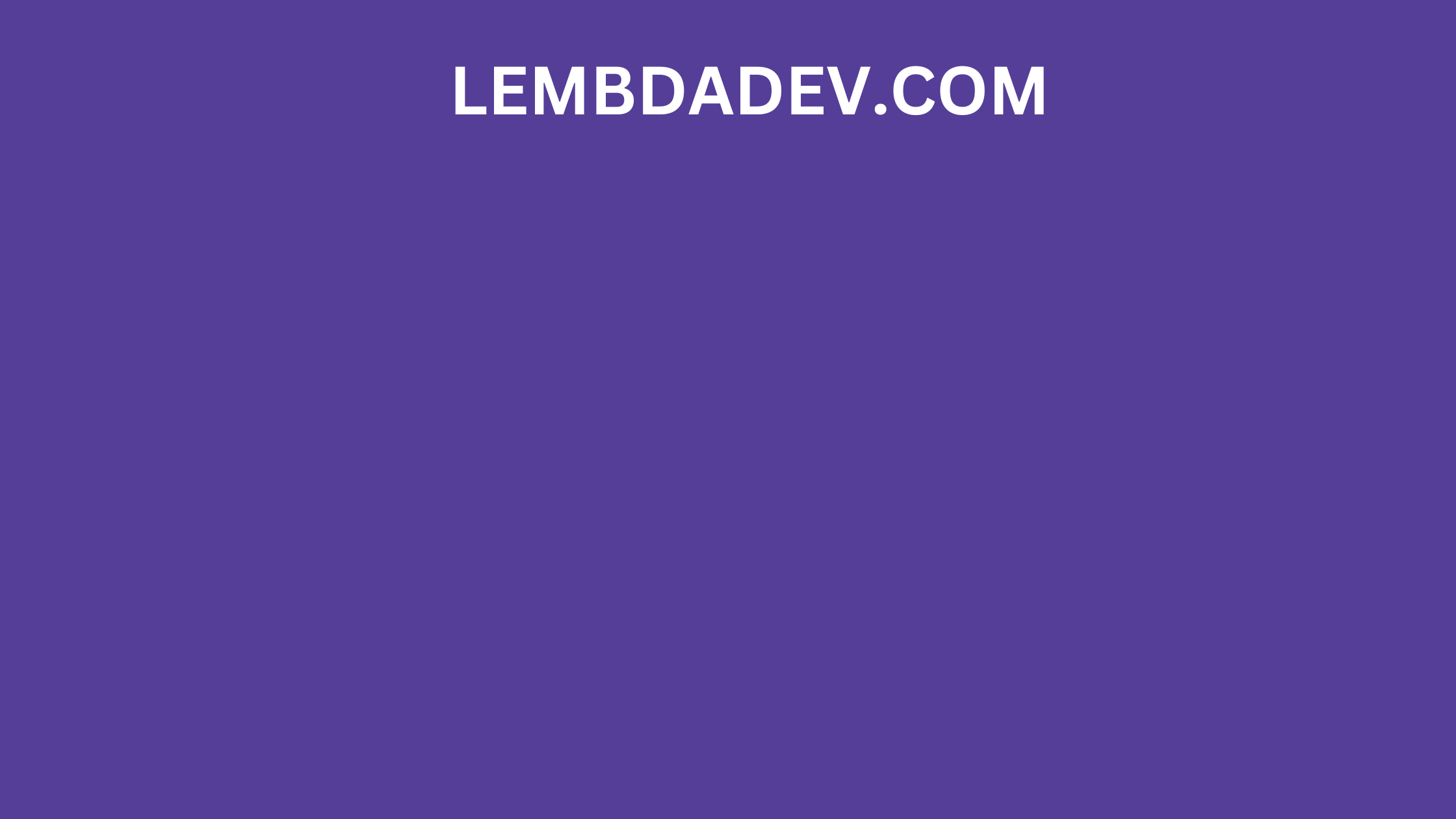Published on

# How to Validate a Date in JavaScript?## How to Validate a Date in JavaScript?

### How to Validate a Date in JavaScript

Validating a date in JavaScript is important to ensure that the data you are using is accurate and consistent. There are a few different ways to validate a date in JavaScript, each with its own advantages and disadvantages.

### 1. Using the Date.parse() method**

The `Date.parse()` method is the simplest way to validate a date in JavaScript. It takes a date string as input and returns the number of milliseconds since the Unix epoch (January 1, 1970, at 00:00:00 UTC). If the date string is invalid, the Date.parse() method returns NaN.

To validate a date using the `Date.parse()` method, you would simply pass the date string to the method and check the return value. If the return value is NaN, the date string is invalid.

### Example of how to validate a date using the Date.parse() method:

``````const dateString = "2023-09-28";

// Validate the date string.
const isValidDate = !isNaN(Date.parse(dateString));

if (isValidDate) {
// The date string is valid.
} else {
// The date string is invalid.
}
``````

### 2. Using regular expressions**

Another way to validate a date in JavaScript is to use regular expressions. Regular expressions are patterns that can be used to match strings of text.

To validate a date using a regular expression, you would first create a regular expression that matches the format of the date string you are trying to validate. Then, you would use the regular expression to match the date string. If the regular expression matches the date string, the date string is valid.

### Example of how to validate a date using a regular expression:

``````const dateString = "2023-09-28";

// Create a regular expression that matches the format of the date string.
const regEx = /^\d{4}-\d{2}-\d{2}\$/;

// Validate the date string.
const isValidDate = regEx.test(dateString);

if (isValidDate) {
// The date string is valid.
} else {
// The date string is invalid.
}
``````

### 3. Using a date library**

There are a number of date libraries available for JavaScript that can be used to validate dates. These libraries typically provide a variety of features, such as the ability to validate dates in different formats, support for different time zones, and the ability to calculate date differences.

To validate a date using a date library, you would simply instantiate the library and then use the library's methods to validate the date.

Here is an example of how to validate a date using the Moment.js library:

``````import moment from "moment";

const dateString = "2023-09-28";

// Validate the date string.
const isValidDate = moment(dateString).isValid();

if (isValidDate) {
// The date string is valid.
} else {
// The date string is invalid.
}
``````

### Conclusion

The best method to use for validating a date in JavaScript depends on your specific needs. If you need a simple and straightforward way to validate a date, then you should use the Date.parse() method. If you need a more flexible way to validate a date, or if you need to support features such as different date formats and time zones, then you should use a regular expression or a date library.